Courses

# Test: Basic Proportionality Theorem

## 5 Questions MCQ Test Mathematics (Maths) Class 10 | Test: Basic Proportionality Theorem

Description
This mock test of Test: Basic Proportionality Theorem for Class 10 helps you for every Class 10 entrance exam. This contains 5 Multiple Choice Questions for Class 10 Test: Basic Proportionality Theorem (mcq) to study with solutions a complete question bank. The solved questions answers in this Test: Basic Proportionality Theorem quiz give you a good mix of easy questions and tough questions. Class 10 students definitely take this Test: Basic Proportionality Theorem exercise for a better result in the exam. You can find other Test: Basic Proportionality Theorem extra questions, long questions & short questions for Class 10 on EduRev as well by searching above.
QUESTION: 1

### In the given fig, P and Q are points on the sides AB and AC respectively of Δ ABC such that AP = 3.5 cm, PB = 7 cm, AQ = 3 cm and QC = 6 cm. If PQ = 4.5 cm, find BC.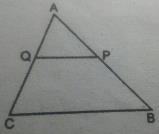Solution: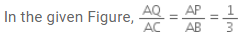Therefore, by converse of Basic Proportionality Theorem, we have QP || CB
Hence, ∆AQP ~ ∆ACB [Using AA similar condition]

∴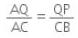⇒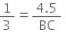⇒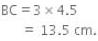QUESTION: 2

Solution:
QUESTION: 3

### ΔABC ~ ΔPQR, ∠B = 50° and ∠C = 70° then ∠P is equal to​

Solution:

Similar triangles have corresponding angles equal. So Angle Q=Angle B = 50° and Angle R = Angle C = 70° . So by angle sum property, Angle P+Angle Q +Angle R = 180°
Angle P=180° - 50° - 70° = 60°

QUESTION: 4

Two congruent triangles are actually similar triangles with the ratio of corresponding sides as.​

Solution:
QUESTION: 5

Which geometric figures are always similar?​

Solution:

It can be found that circles map one  onto another.So they are similar figures. A regular polygon is a polygon which has the same sides and equal measures of angles. So they are also similar.